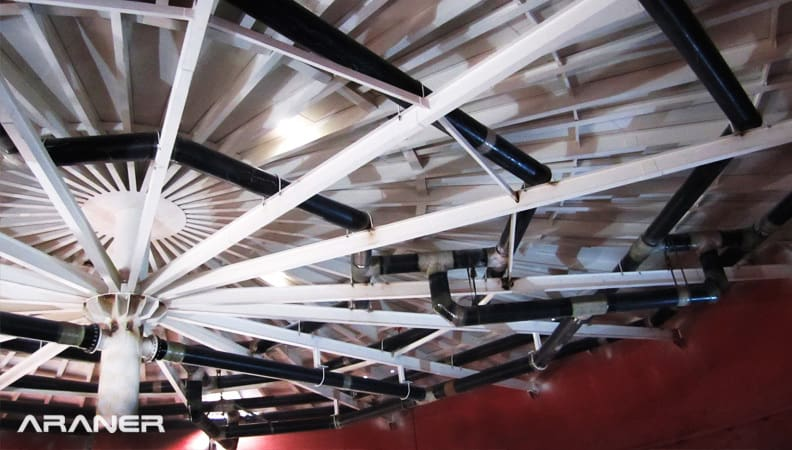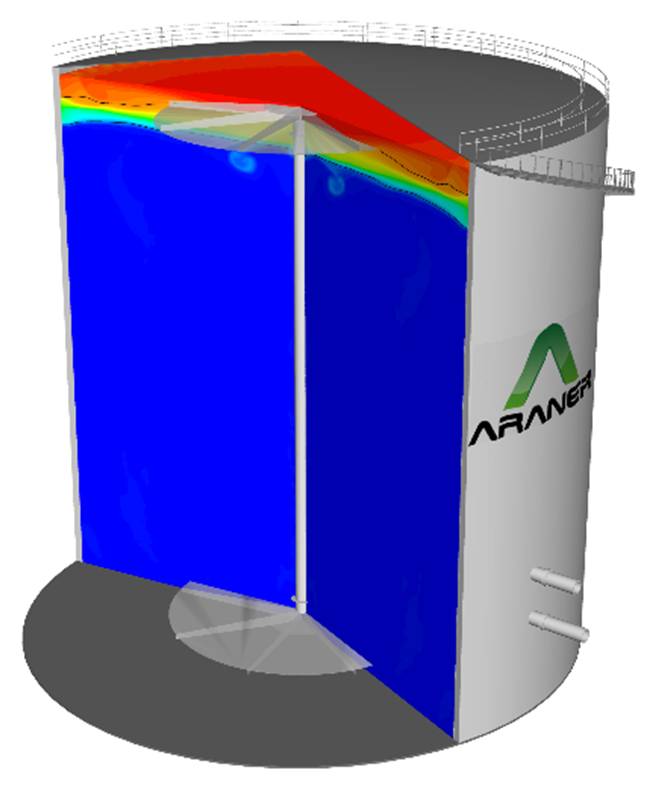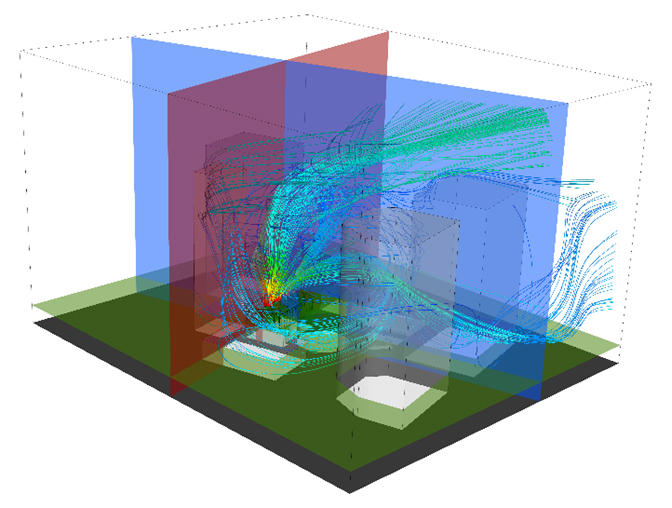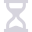Thermal Energy Storage (TES) is a technology which allows the storage and transfer of thermal energy, either heat or cold. TES takes advantage of off-peak periods of electrical demand to produce thermal energy, which is accumulated in the tank for later use during peak periods. Hot or chilled water enters and leaves the Thermal Energy Storage tank through diffusers which prevent turbulence in the tank and allow the water to stratify. Layers of chilled water are formed at the base of the tank and layers of hot water at the top, with a sharp and narrow transition layer called thermocline.Figure 1: Inside of a Thermal Energy Storage Tank

When a TES system is implemented in a District Cooling plant, it takes advantage of off-peak hours to use the chiller to create chilled water that wouldn’t normally be necessary and stores it. The next day, the chilled water that has been stored in the tank is used to augment the chilling provided by the direct cooling plant during times of peak demand. The addition of TES allows cooling equipment to be sized for average loads instead of peak loads, meaning smaller equipment and lower costs. TES also maximizes efficiency by simultaneously producing chilled water for storage while meeting off-peak demands. ARANER TES systems are customized to project specifications using Computational Fluid Dynamics (CFD) to model the systems inflow and outflow as well as the behavior of the thermocline. CFD involves the use of physics, applied mathematics, and numerical models to visualize the flow of liquids or gases and the variation of their thermo-fluid properties throughout its motion.## Computational Fluid Dynamics

Computational fluid dynamics is based on the Navier-Stokes equations which describe the fluid flow. These equations explain how the velocity, pressure, temperature, and density of a moving fluid are related. The equation, also called the momentum equation, is found by applying Newtons Second Law of motion, force is equal to mass times acceleration (F=ma), to a fluid element. The Navier-Stokes equation must be used in conjunction with the continuity equation which accounts for the mass conservation of flow, and the energy equation which accounts for the conservation of energy; the equations together are often referred to as the Navier-Stokes Equations. They are partial differential equations that describe the balance of the forces at a given point within a fluid.Figure 2: Detailed analysis of the thermocline formation during the discharging phase of a TES tank

Computational Fluid Dynamics represents the optimal technique for evaluating how a TES system will function once it is built since it allows an overview of the effects of flow and temperature. Thus, we can check how much cooling will be available with a given TES configuration. Computational fluid dynamics models fluids on a continuum, it assumes that the fluid completely fills the space.  The computational software allows for consideration of the geometry of the system, the volume of fluid contained in the system, and boundary conditions which exist at points through the system are also considered. Discover our 1-minute video of a CFD simulation in a TES Tank. To analyze a system using CFD, the geometry of the system must first be established using Computer-Aided Design (CAD) tools, and the fluid field is extracted. The volume occupied by the fluid field is then broken down into a mesh of distinct cells, and the physical modeling is defined. It is important to note that the size of the mesh selected can affect the model, too small and the model will take a long time to calculate, too large and the model may be less accurate than it would be with a smaller mesh. The user must also enter the boundary conditions, which are a series of assumptions, such as slip-free walls where velocity is zero, inlet conditions, outlet conditions, the use of insulation in walls, and other conditions which can affect flow and temperature. The computer uses the information which is known about the fluid and the system to develop a mathematical model of the calculated physical properties of the system at any given point in the system or time. The model describes the changes to all the physical properties of the systems fluid flow and heat transfer.Figure 3: Assessment of the effects of surrounding buildings on the performance of cooling towers in urban environments.

In particular, the investigation of fluid flow with thermal changes depends on simultaneously solving the equations for velocity, pressure, and temperature. However, the final form of the Navier-Stokes equations also contains four other thermodynamic variables; density, enthalpy, viscosity, and thermal conductivity. The use of additional variables allows the computer to use the known physical properties of the fluid, the geometry of the system, and the boundary conditions to solve the equations for the entire system and across a given period of time, usually a one-day temperature cycle in the case of TES. The result is a true indication of how a system can be expected to function, telling us such important factors as how long it will take for the stored thermal energy to be fully utilized. These programs can generate a snapshot in time of the system, or a component thereof, in time allowing us to visualize physical parameters such as temperature. The computer can even create a colored representation of the system showing where the system will be cold and where it will be warm, in the case of temperature, for example. The computer model can thus be used to determine the full impact that TES will have on a District Cooling plant and the information used to size the equipment to provide the flows necessary after the augmentation of cooling from the energy stored in the TES.

## Thermal Energy Equation Physics: Conclusion

CFD techniques used to conduct TES analysis is key in accurately predicting the physical properties of a proposed system. It is also important that those running CFD models are knowledgeable regarding the technique, the physics of the problem and the characteristics of the system. ARANER has the knowledge and experience necessary to develop an accurate model of the physical properties of your system, so contact us today to learn more. To discover more about Computational Fluid Dynamics and Thermal Energy Storage, discover our high-level ebook.5 min